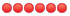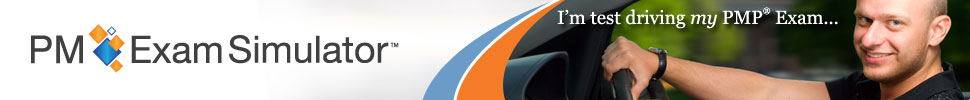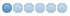NEVER post copyrighted questions. Always state where questions come from. We take copyright violations very serious. Any copyrighted materials will be removed and the posters account will be deactivated.
 Page:1

TOPIC: Free PMP® Exam Sample Question

Free PMP® Exam Sample Question 2 months 4 weeks ago #28546

 Mary Kathrine PaduaTopic Author Offline AdministratorPosts: 237 Karma: 10 Thank you received: 107You are managing a renovation project of a house which is to be completed at a budget of \$100,000. Earned value analysis shows that so far you have completed 40% of the work and spent \$60,000. How much more will it cost to complete the project if the cost performance remains unchanged? A. \$160,000 B. \$149,254 C. \$120,000 D. \$89,254 HINT: Determining how much more it will cost to finish the project is the ETC. Also, consider the cost performance, which will remain unchanged. All our questions are updated to the latest A Guide to the Project Management Body of Knowledge (PMBOK® Guide) standard. Stop by at free.pm-exam-simulator.com/ and try the PM Exam Simulator free for 7 days. We are a trusted and experienced education provider. Answer and Explanation: The correct answer is D. The total budget, or budget at completion (BAC), is \$100,000. The cost so far, or actual cost (AC), is \$60,000. You have completed 40% of the work which is the earned value (EV), i.e., EV = 40% x \$100,000 = \$40,000. Understanding what exactly the question asks is the key to answering the question correctly. The last sentence of the question starts with "How much more will it cost to complete the project...", implying we are requested to find the estimate to complete (ETC). The ETC can be calculated as follows: ETC = EAC - AC, where the EAC is the estimate to complete. Selecting the correct formual for the EAC is another key to answering this question correctly. The last sentence ofthe question ends with "...if the cost performance remains unchanged?", implying that the formula for the EAC should consider the cost performance index (CPI), i.e.: EAC = BAC / CPI The CPI, in turn, can be calculated using the following formula: CPI = EV / AC = 40,000 / 60,000 = 0.67 The rest of the calculations are shown below: EAC = 100,000 / 0.67 = 149,254 ETC = 149,254 - 60,000 = 89,254 Therefore, the cost to complete the renovation if the cost performance remains unchanged is \$89,254 Details for each option: A. Incorrect. This answer choice does not represent a result of a specific formula used in the earned value analysis. We have obtained this number by adding \$100,000, which is the budget at completion (BAC), to \$60,000, which is the actual cost (AC). B. Incorrect. The value in this answer choice represents the EAC which has been calculated using the formula: EAC = BAC / CPI, while the question is asking to determine the cost to complete the renovation or ETC. C. Incorrect. The value in this answer choice represents the EAC which has been calculated using the formula: EAC = AC + BAC - EV, while the question is asking to determine the cost to complete the renovation or ETC. D. Correct. The cost required to complete the project is \$89,254. The value was derived using ETC = EAC - AC utilizing the formula EAC = BAC / CPI to calculate EAC. Reference: A Guide to the Project Management Body of Knowledge (PMBOK® Guide) – Sixth Edition, Project Management Institute Inc., 2017, Page(s) 267 The following user(s) said Thank You: Rony Crevecoeur Your e-mail address will never be displayed on the site. Check this box to be notified of replies to this topic. Note: BBcode and smileys are still usable.

Free PMP® Exam Sample Question 2 months 3 weeks ago #28558

 Derek LudlowOffline Fresh BoarderPosts: 1 Thank you received: 0 This answer contains a rounding error. The correct answer is \$90,000, but because you used 0.67 as a proxy for 2/3, your answer was slightly different. In the actual exam I never saw answers with rounding errors. Does that happen? That being said, for students looking to get good marks should seek answers close to what they calculated if they are confident in their calculations. Your e-mail address will never be displayed on the site. Check this box to be notified of replies to this topic. Note: BBcode and smileys are still usable.
 Page:1
Moderators: Yolanda MabutasMary Kathrine PaduaJoe PangJohn Paul BugarinLisa Sweeney PMPBrad Pennington, PMPKyle Kilbride, PMP

OSP INTERNATIONAL LLC
OSP INTERNATIONAL LLC
Training for Project Management Professional (PMP)®, PMI Agile Certified Practitioner (PMI-ACP)®, and Certified Associate in Project Management (CAPM)®